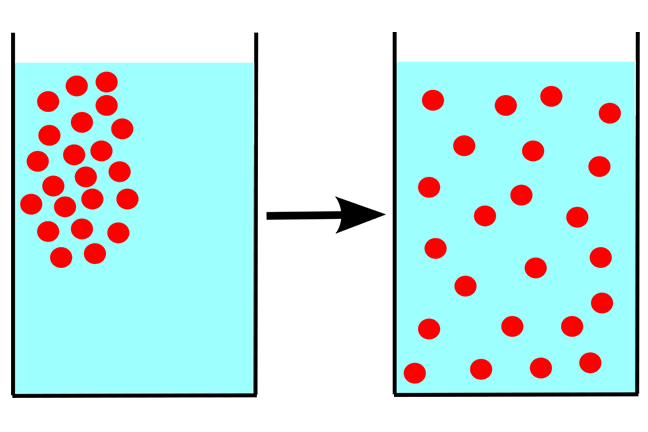1.11

## UNSW SydneyDiffusion

# Boyle's law and the Ideal Gas law

Boyle’s law is a fundamental relation between the pressure and the volume of a gas. It is also a great example of an inverse relation.

In this step we will learn

• about Boyle’s law and its generalisation the Ideal Gas law

• what happens to a helium balloon after you let it go

## Boyle’s lawThe Shannon Portrait of the Hon Robert Boyle Chemical Heritage Foundation, CC BY 3.0, via Wikimedia Commons

Robert Boyle in 1662 published his observations of a law that actually had been discovered earlier by Richard Towneley and Henry Power. Boyle was interested in the properties of air, and by subjecting a cylinder of air to various pressures by using mercury, he was able to verify that indeed the $\normalsize{\operatorname{volume}}$ was inversely proportional to the $\normalsize{\operatorname{pressure}}$. So

or equivalently

Here is a copy of Boyle’s original observations.Plot of data points for Boyle’s Law, revealing an inverse relation By Krishnavedala (Own work) CC BY-SA 3.0, via Wikimedia Commons

In fact this law only holds if the temperature of the gas is held fixed.

Q1 (E): Boyle’s original experiments used the units inches of mercury to measure pressure (there is a direct proportionality between these units and other, more familiar, units). If Robert Boyle compressed the volume of gas to 1/10th the original volume, what happened to the pressure?

## The volume of a balloon

Suppose we fill a balloon with helium and let it go. Naturally it goes up. As it goes up, the air pressure decreases, and so according to Boyle’s law, the volume will increase. In this next question, you can grapple with some specific numbers, but we will not worry too much about the exact definitions of the units involved!

Q2 (M): Suppose a balloon is filled with helium, and then it ascends from sea level, where the atmospheric pressure is $\normalsize{101.325}$ kPa (the unit is called the kilo Pascal: where a Pascal is a Newton per square meter), to an altitude of $\normalsize{0.6}$ kilometres. What has happened to its volume at this point? (to answer this read the remarks below)

To answer this, you need to know that air pressure decreases approximately linearly by about $\normalsize{12}$ kPA per kilometre for the first kilometre or two. In fact here is the relation between altitude and air pressure, using a graphical relation:Atmospheric Pressure vs Altitude By Geek.not.nerd (Own work) [CC0], via Wikimedia Commons

You might also like to ignore changes of temperature. In fact, however, the temperature also decreases as you gain altitude, at least till you get to the end of the troposphere (the lower part of the atmosphere in which most our usual weather takes place). Moreover this is roughly also a linear change!

Q3 (M): If the surface temperature at sea level is $\normalsize{30}$ degrees (C), and the temperature decreases linearly to $\normalsize{-50}$ degrees at an altitude of $\normalsize{10}$ kilometers, then what will be the temperature at an altitude of $\normalsize{0.6}$ kilometers?

## The Ideal Gas law

In fact, Boyle’s law needs to be modified if temperature changes become significant. This was discovered $\normalsize{200}$ years later. The Ideal Gas law is a generalization of Boyle’s law, and also includes as special cases two other laws called Charles’s law and Avogadro’s Law. It was discovered by E. Clapeyron in 1834 and contains a lot of variables, along with a constant of nature $\normalsize R$ whose value depends on the units chosen.

The Ideal Gas law is:

where: $\normalsize{P}$ is pressure, $\normalsize{V}$ is volume, $\normalsize{n}$ is the total amount of gas, $\normalsize{R}$ is a constant and $\normalsize{T}$ is absolute temperature.

The law applies to an amount $\normalsize{n}$ of an ideal gas in a container of some volume $\normalsize{V}$, at some temperature $\normalsize{T}$ and with some pressure $\normalsize{P}$. It is easier to understand if we look at the relation between two quantities at a time.

Q4 (E): Assuming the other quantities are constant, what kind of relation do we have between the temperature and pressure of a gas at a constant volume? What is the meaning of this physically?

Q5 (H): Returning to our helium filled balloon, what is the change of volume from sea level to an altitude of $\normalsize{0.6}$ kilometres, if we now consider both the change in atmospheric pressure and temperature? (You can use the answers of Question 2 and Question 3)

## Answers

A1. If the volume decreases to one-tenth its original value, then correspondingly the pressure must be multiplied by $\normalsize{10}$ in order that the product of volume and pressure be the same as before.

A2. When the altitude changes by $\normalsize{0.6\;\text{km}}$, the pressure decreases by $\normalsize{7.2 \;\text{kPa}}$, and so the pressure at the altitude of $\normalsize{0.6\;\text{km}}$ is $\normalsize{94.125\;\text{kPa}}.$ By Boyle’s law the volume of the balloon at sea level, denoted by $\normalsize{V_0}$, and the volume at the altitude of $\normalsize{0.6\;\text{km}}$, denoted by $\normalsize{V_1}$, are related by the relation $101.325\;V_0=94.125\;V_1$. Thus, in proportion to the original volume, the final volume is

which means that the volume has increased.

A3. One possible way to solve this problem is to find the (linear) equation of the temperature $\normalsize{T}$ in terms of altitude $\normalsize{y}.$ One can obtain that the equation is $\normalsize{T=30-8y}.$ Therefore, for the altitude $\normalsize{y=0.6\;\text{km}}$ we have that $\normalsize{T=30-8\times 0.6=25.2}$ degrees (C).

Importantly, if we want to use these temperatures in the Ideal gas law we must convert our temperatures to an absolute temperature scale, like degrees Kelvin. In Kelvin these temperatures are $\normalsize 273.15+30=303.15$ degrees (K) at sea-level and $\normalsize 273.15+25.2=298.35$ degrees (K) at $\normalsize{0.6\;\text{km}}$.

A4. The relation between temperature and pressure is a direct proportionality given by

The physical meaning is that as the temperature increases, the pressure also increases (and vice versa).

A5. The ideal gas law can be re-arranged as follows.

but we know that $\normalsize{nR}$ is constant because the amount of the gas in the balloon does not change, therefore we must have that

from which we can see that